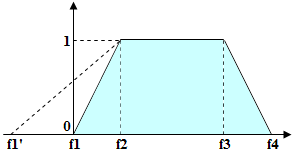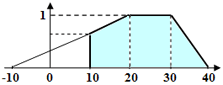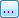English (English)

# Fuzzy interval

A fuzzy interval is a tool enabling the program to assess the quality of hypothesis based on its length. A fuzzy interval may be measured in units of length (dots, millimeters, etc.) or in characters (in the case of lines). For a fuzzy interval, four values must be specified which define the optimal and possible ranges of values.

Suppose you have a fuzzy interval [f1,f2,f3,f4] and the length of the detected string of characters (or the length of the detected space) is L. If the length L is in the range from f2 to f3 (i.e. L>=f2 and L<=f3), the quality of the hypothesis is 1. If the length is in the range from f1 to f2, the quality of the hypothesis changes in direct proportion from 0 to 1 (Quality(f1) = 0, Quality(f2) = 1). Similarly, if the length is in the range from f3 to f4, the quality of the hypothesis changes in direct proportion from 1 to 0 (Quality(f3) = 1, Quality(f4) = 0). If the length does not fall in the range from f1 to f4 (i.e. Lf4), the quality of the hypothesis is 0 (Quality(L) = 0). The quality of the hypothesis for the detected object is multiplied by the value of the Character count property. The value of this property is selected depending on the length of the detected object.Note.The quality of any chain of hypotheses for several elements is calculated by multiplying the hypotheses for each element. If the chain is relatively long and the quality estimates of the constituent hypotheses are too low as a result of too strict constraints, the resulting quality of the entire chain may be too low.

Make sure that the selected hypothesis has the biggest quality estimate possible. On the other hand, you need to be able to distinguish hypotheses by their qualities, so as to be able to select the best one. Therefore, you need to set up fuzzy intervals so that acceptable hypotheses are not penalized too much.

You can also use negative values for the left boundary of the fuzzy interval (even though in reality there are no strings of negative length). This may be useful, as it will make the dependence of quality in the range from 0 to 1 less steep, thereby reducing the penalty. If at the same time you need to set the bottom limit for this parameter (e.g. the length of the string cannot be smaller than 10 characters and the fuzzy interval for the length of the string is [-10,20,30,40]), you can do so directly on the Advanced tab by typing Value.Length >= 10 in the Advanced post-search relations pane.In general, it is advisable not to set too rigid intervals. This is particularly important when processing images of varying quality. On some images for example, there may be spaces within letters due to poor quality of the source document or bad scanning options. In this case the program may interpret one character as several characters, which may lead to a drastic reduction of the quality of the hypothesis if the fuzzy interval was too rigid. As a result, the program will discard a hypothesis which is in essence correct and select another hypothesis. For this reason, if you need to select between Character String hypotheses by comparing their lengths, you would do best by specifying additional constraints in the Advanced post-search relations pane.

### Fuzzy interval visual editor

ABBYY FlexiLayout Studio offers a visual editor to make specifying fuzzy intervals easier. You can open the fuzzy interval editor from the Properties dialog box of a Character String element (Character String tab,buttons) or from the main menu by selecting Tools → Fuzzy Interval Editor....

25.05.2023 7:55:03